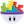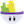Howdy, Stranger!

It looks like you're new here. If you want to get involved, click one of these buttons!

display y position without numbers after the ,

Posts: 2,313Memberhow can you display the texts of a constrained y position without numbers after the ,
hope I explained enough
thanks✮ Available for hire! [email protected] ✮

• Posts: 334Member• Posts: 2,313MemberI don't really understand what you mean

✮ Available for hire! [email protected] ✮

• Posts: 2,313Memberthen there is still 1 number behind the ,

✮ Available for hire! [email protected] ✮

• Posts: 2,313Memberoh no there is not sorrythere was still standing /10 hahathanks this worked

✮ Available for hire! [email protected] ✮

• London, UK.Posts: 12,822Member@timolapre1998

. . . . or another way:

floor( self.Position.Y )
• Posts: 2,313Memberthanksis there a tutorial where I can see what all the functions like floor padint abs and acos do?

✮ Available for hire! [email protected] ✮

• London, UK.Posts: 12,822Memberedited June 2013
@timolapre1998

abs

Usage: abs(x)

The absolute value of the number. i.e. make the number positive if it is negative.

Examples:

abs(-42) = 42

abs(42) = 42

Usage Example: Combined with a cosine or sine function, you can make an actor “hop” in a pattern.

. . . . . . . . . .

acos

Usage: acos(x)

The trigonometric arccosine (inverse cosine) function. Values can only range from -1 to 1 with results from 180 to 0, respectively. Any other input values will result in ‘nan’.

. . . . . . . . . .

asin

Usage: asin(x)

The trigonometric arcsine (inverse sine) function. Values can only range from -1 to 1 with results from -90 to 90, respectively. Any other input values will result in ‘nan’.

. . . . . . . . . .

atan

Usage: atan(x)

The trigonometric arctangent (inverse tangent) function. Results will range from -90 to 90.

. . . . . . . . . .

ceil

Usage: ceil(x)

The integer when you round up a value. e.g. ceil(0) = 0 , ceil(0.004) = 1

. . . . . . . . . .

cos

Usage: cos(x)

The trigonometric cosine function. If the value that you use the cosine function on starts at 0, your resulting value will start at the maximum value of cosine. i.e. cos(0) = 1. Check out other possibilities of sine and cosine at http://en.wikipedia.org/wiki/Trigonometric_functions

. . . . . . . . . .

exp

Usage: exp(x)

The exponential function, e^x where e is the number (approximately 2.718281828).

Check out other possibilities at http://en.wikipedia.org/wiki/Exponential_function

. . . . . . . . . .

floor

Usage: floor(x)

The integer when you round down a value.

Example: floor(0) = 0 , floor(0.004) = 0

. . . . . . . . . .

ln

Usage: ln(x)

The natural logarithm of a value. The natural logarithm is the logarithm to the base e, where e is an irrational constant approximately equal to 2.718281828.

Check out other possibilities at http://en.wikipedia.org/wiki/Natural_logarithm

Example: ln(e) = 1

. . . . . . . . . .

log10

Usage: log10(x)

The base 10 logarithm of a value.

Check out other possibilities at http://en.wikipedia.org/wiki/Logarithm

Example: log10(10) = 1, log10(100) = 2

. . . . . . . . . .

magnitude

Usage: magnitude(x,y)

Find the length of a line segment from the origin, (0,0), to the given point. You can include an offset,(x',y'), to find the length between the two points. i.e. magnitude(x-x',y-y')

Example: If I wanted to find the length between my actor at coordinates (25,30) and another actor at coordinates (100,240), I would use the expression magnitude(25-100,30-240).

. . . . . . . . . .

max

Usage: max(x,y)

Return the higher value of two numbers or variable values.

. . . . . . . . . .

min

Usage: min(x,y)

Return the smaller value of two numbers or variable values.

Example: min(12,35) = 12

. . . . . . . . . .

pow

Usage: pow(x,y)

Return the value of x to the power of y.

Example: power(2,3) = 222 = 8

. . . . . . . . . .

random

Usage: random(x,y)

Generate a random integer equal to or between the first integer value and the second integer value.

Example: random(1,10) can be any number from the set of {1,2,3,4,5,6,7,8,9,10}.

. . . . . . . . . .

sin

Usage: sin(x)

The trigonometric sine function. This is similar to the cosine function but it is offset by one quarter of a wave cycle.

Tip: If you use the sine function and start your incrementing variable at 0(using the example above), your actor’s movement does not start at the middle between the minimum and maximum points of the wave.

. . . . . . . . . .

sqrt

Usage: sqrt(x)

Square root of a value. Input values less than 0 will result in ‘nan’.

. . . . . . . . . .

tan

Usage: tan(x)

The trigonometric tangent function.

. . . . . . . . . .

vectorToAngle

Usage: vectorToAngle(x,y)

Find the angle relative to the origin, (0,0), given an X and Y coordinate. You can include an offset,(x',y'), to find an angle relative to the offset.

e.g. vectorToAngle(x-x',y-y')
This discussion has been closed.